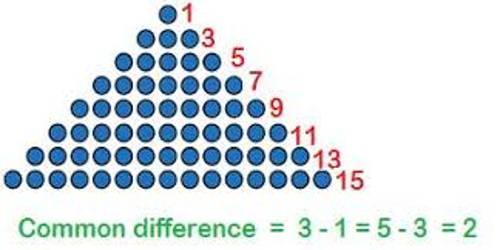Arithmetic Progression

In mathematics, an arithmetic progression (AP) or arithmetic sequence is a sequence of numbers such that the difference between the consecutive terms is constant. It is a sequence of numbers in which the consecutive terms (beginning with the second term) are formed by adding a constant quantity with the preceding term.

Definition of Arithmetic Progression: A sequence of numbers is known as an arithmetic progression (A.P.) if the difference between the term and the preceding term is always same or constant.

An arithmetic progression is a sequence of numbers such that the difference between any two successive members is constant.

• For example, the sequence 1, 2, 3, 4, … is an arithmetic progression with common difference 1.
• Second example: the sequence 3, 5, 7, 9, 11,… is an arithmetic progression with common difference 2.
• Third example: the sequence 20, 10, 0, -10, -20, -30, … is an arithmetic progression with common difference -10.

The constant quantity stated in the above definition is called the common difference of the progression. The constant difference, generally denoted by d is called common difference.

an+1 – an = constant (=d) for all n ∈ N

From the definition, it is clear that an arithmetic progression is a sequence of numbers in which the difference between any two consecutive terms is constant.Broad Explanations on Arithmetic Progression:

(1) -2, 1, 4, 7, 10 ……………. is an A.P. whose first term is -2 and common difference is 1 – (-2) = 1 + 2 = 3.

(2) The sequence {3, 7, 11, 15, 19, 23, 27, …………………} is an Arithmetic Progression whose common difference is 4, since

Second term (7) = First term (3) + 4

Third term (11) = Second term (7) + 4

Fourth term (15) = Third term (11) + 4

Fifth term (19) = Fourth term (15) + 4 etc.

(3) The sequence {58, 43, 28, 13, -2, -17, -32, …………………} is an Arithmetic Progression whose common difference is -15, since

Second term (43) = First term (58) + (-15)

Third term (28) = Second term (43) + (-15)

Fourth term (13) = Third term (28) + (-15)

Fifth term (-2) = Fourth term (13) + (-15) etc.

(4) The sequence {11, 23, 35, 47, 59, 71, 83, …………………} is an Arithmetic Progression whose common difference is 4, since

Second term (23) = First term (11) + 12

Third term (35) = Second term (23) + 12

Fourth term (47) = Third term (35) + 12

Fifth term (59) = Fourth term (47) + 12 etc.

An algorithm to determine whether a sequence is an Arithmetic Progression or not when its nth term is given:

Step I: Obtain an

Step II: Replace ‘n’ by n + 1 in an to get an+1.

Step III: calculate an+1 – an.

When an+1 is independent of n then, the given sequence is an Arithmetic Progression. And, when an+1 is not independent of n then, the given sequence is not an Arithmetic Progression.

Information Source: